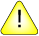This version of this document is no longer maintained. For the latest documentation, see http://www.qnx.com/developers/docs.

# drem(), dremf()

Compute the remainder of two numbers

## Synopsis:

```#include <math.h>

double drem ( double x,
double y );

float dremf ( float x,
float y );```

## Arguments:

x
The numerator of the division.
y
The denominator.

## Library:

libm

Use the -l m option to qcc to link against this library.

## Description:

The drem() and dremf() functions compute the remainder r = x - n * y, when y is nonzero. The value n is the integral value nearest the exact value x/y.

When |n - x/y| = 1/2, the value n is chosen to be even. But remainder(x, 0) and remainder(infinity,0) are invalid operations that produce a NAN.

The behavior of drem() is independent of the rounding mode.

## Returns:

The remainder, r = x - n * y, when y is nonzero.

## Classification:

Unix

Safety:
Cancellation point No
Interrupt handler No
Signal handler No